# Dynamic Programming with Ruby

Ruby

Share:

#### Free JavaScript Book!Write powerful, clean and maintainable JavaScript.

RRP \$11.95

The study of algorithms forms a cornerstone of computer science research and education. However, with our web-centered community, Rubyists are often unaware of common algorithmic techniques.

This article will give you a taste of a common algorithm design technique called “dynamic programming” by exploring two different problems and presenting solutions in Ruby. It turns out that dynamic programming is actually a very versatile approach that can be applied to all sorts of problems in a ton of disciplines.

A word of warning: If this is your first exposure to algorithms with some mathematical flavor, the going might seem a bit difficult. But, I highly encourage you to keep at it! The feeling that you will get once it “clicks” is awesome. If you have any questions, please drop them in the comments below.

Let’s jump in!

## What is it?

First of all, the name “dynamic programming” has absolutely nothing to do with the practice of writing code. It actually started out as a method to solve complicated mathematical problems. In particular, dynamic programming (referred to as DP) is often defined as a method that solves a large problem by breaking it into successively smaller problems. That is a bit vague and for good reason: Since DP can be applied in so many different ways, the underlying concepts are quite general. The best way to really understand something is to use it, so let’s check out a problem we can solve with DP.

## Longest Increasing Subsequences

Let’s say we have a sequence of numbers. We can define a subsequence of this sequence as any subset of the numbers taken in order that they are in the sequence. We can say that a subsequence is increasing if every element is larger than the ones that come before it.

Here’s the problem: Find the length of the longest increasing subsequence of a sequence A.

Alright, let’s try to get a feel for the problem before we attempt to put together a solution. Say we’re given the following sequence:

``seq = [2, 9, 8, 4]``

The sequence itself is not increasing. Let’s take a look at the subsequences of length three to see if any of them are increasing:

``````[2, 9, 8]
[2, 8, 4]
[9, 8, 4]``````

Clearly, none of them are strictly increasing. Checking the subsequences of length two, it is pretty clear that some are increasing, e.g.

``[2, 9]``

So, our answer (which is the maximum length of increasing subsequences) should be two. Now, the question is, how do we tell the computer to do this? A naive approach would be to check if the whole sequence was increasing, check if all subsequences of length n-1 are increasing (where n is the length of the sequence) and so on until we hit a length where we can find an increasing subsequence. But, this is is something called an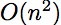algorithm, which means that it scales quadratically in reference to the size of the input sequence.

Can we do better?

Turns out that we can, and we’ll do it by using some DP magic. First of all, how many subsequences do we have that end at `seq`? Just one: the subsequence of length one that contains only `seq`. How about those ending at `seq`? Hmmm… since `seq , we can simply add on ``seq` to the end of the subsequence(s) ending at `seq`. So, the maximum length of a subsequence ending at `seq` is one plus the maximum length of subsequences ending at `seq`, which gives us a maximum subsequence length of two (ending at `seq`).

Let’s try to generalize that. Suppose we want to find out the maximum length of subsequences ending at `seq[j]`. Say we have an array called `q` that stores the indices of sequence elements that come before `seq[j]` and are smaller than `seq[j]`. Taking an example: for `seq = [2, 4, 10, 9, 4]` and `j = 3` (remember, `j` is an index of `seq`), then `q = [2, 4]` since these are the only elements that come before `seq[j]` and are smaller than `seq[j]`.

Then, we can say that the maximum length of increasing subsequences ending at `seq[j]`
is equal to 1 plus the maximum length of the increasing subsequences at all the indices
in `q`. Basically, we’re using things we’ve already computed to figure out
how to come up with a final solution.

The code always helps to make stuff clear:

``````def longest_incr_subseq(seq)
l = {}

seq.length.times do |j|
l_conn_keys = l.keys.select do |i|
i < j and seq[i] < seq[j]
end

l_conn_values = l_conn_keys.map do |k|
l[k]
end

l[j] = 1 + (l_conn_values.max || 0)
end

return l.values.max
end``````

Let’s break it down.

``l = {}``

Here, we define a hash that maps indices to the maximum length of the increasing subsequences that end at that index. Going back to an example, if `seq=[2, 4, 3]`, then `l = {0=>1, 1=>2, 2=>2}`. There’s only one possible subsequence ending at 0: ``; so `l = 1`. We then use the algorithm to figure out the rest
of `l`.

``````seq.length.times do |j|
...
end``````

We’re looping over all the indices in the sequence because we have to fill in a value for all of them in the `l` structure.

``````seq.length.times do |j|
...
l_conn_keys = l.keys.select do |i|
i < j and seq[i] < seq[j]
end
...
end``````

`l_conn_keys` is equivalent to the `q` we discussed earlier. It contains the indices that come before `j` and have values smaller than that of `j`. This implies that increasing subsequences that end in an element of `l_conn_keys` can be extended into a longer increasing subsequence by simply adding `seq[j]` to the end.

``````seq.length.times do |j|
...
l_conn_values = l_conn_keys.map do |k|
l[k]
end
...
end``````

We are taking `l_conn_keys` (which is a list of indices for `seq`) and converting it into a list of values from the `l` Ruby hash. That gives us the maximum length of increasing subsequences at all of the connecting indices.

``l[j] = 1 + (l_conn_values.max || 0)``

Here’s the heart of the algorithm. We say that we can add the our element (extending the length by 1) to the length associated with the index that has the highest max length subsequence.

Let’s work through an example. Say `seq = [2, 4, 9, 5]`. For `j=0`, it is pretty easy to see that `l_conn_keys = []`. Thus, `l_conn_values = []`. Then, with the expression `l[j] = 1 = (l_conn_values.max || 0)` gives us `l = 1`. Set `j=1`. Remember that `l_conn_keys` is the indices that “connect” to `j=1`, so we get `l_conn_keys = ` which then translates to `l_conn_values = `. We get `l = 1 + 1 = 2`. Similarly, we can consider `j=2` to get `l = 3`. Now `j=3` is a little bit more interesting. Notice that `l_conn_keys = [0, 1]` since 9 (index of 2 in the sequence) is greater than 5. Then we get `l_conn_values = [1, 2]`, finally getting `l = 2 + 1 = 3`.

That gives us the correct algorithm (the proof itself is actually quite simple and uses mathematical induction – bonus points if you can write it up!). Let’s think a little bit about how it performs. We’re iterating over the whole sequence only once (or a constant multiple of “once”) so we can say that the algorithm is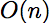or that it scales linearly. Think about in comparison to our naive algorithm which was quadratic. This difference can be enormous at scale!

Out of the whole nitty-gritty of the problem, the take-home points are:

• We broke a large problems into smaller problems then built the large solution from the small solutions
• We used the maximum length of the subsequences ending at a certain index. This “ending at a certain index” trick is actually very useful in all sorts of problems.
• Our algorithm was quite a bit faster than the algorithm we reached for at first

## Department Store

This time, instead of dropping into Ruby-speak right-away, we’ll try to use some math in describing our algorithm. In many cases, doing so can make arguments more clear.

Say you run a department store chain. You have a certain location (called Location 0) from which you measure distances along a straight road. You are given a list of possible locations for stores as distances from Location 0 as a vector/set: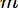For each location in m there is a corresponding profit, which are listed in p with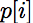corresponding to the locationAnd, you are also given a numberYou are not allowed to set up two stores that are less than k miles apart. The problem is simple: How do you maximize your profit?

Holy cow, that seems really complicated. You have to somehow juggle between the distance requirement and the stores with the highest profits. Turns out that we can use dynamic programming to get a solution to this problem!

Let’s define a function (in the mathematical sense) T where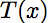represents the maximum profit if you were to just end the list of stores at distance x. So, the value we are looking for at the end of the day is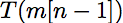where n is the number of stores.

First of all, notice that the maximum profit is unchanged for distances between stores. For example, if we have a store at 10 and another at 20: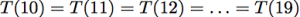Now, let’s try to break down the bigger problem of computinginto some smaller problems. Say we want to maximize profit up to the distancefor some i. Then we can include all of the stores that are k units “behind”So, we can write: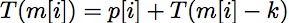Also, if we end our distance right at the first store then the maximum profit is the profit that the first store can attain. In math: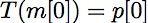Now that we have the mathematical idea behind the DP algorithm, let’s code it up in Ruby:

``````def T(x, m, t_hash)
return 0 if x < m

keys = t_hash.keys.sort
relative_distances = keys.map do |d|
x - d
end

#filter out the stores to leave only the
#ones that come atleast "k" units before "x"
filtered_distances = relative_distances.select do |r|
r >= 0
end

key = keys[filtered_distances.index filtered_distances.min]
return t_hash[key]
end

def dept_store(m, p, k)
t_hash = {m => p}

(1..m.length-1).to_a.each do |j|
b = m[j] - k
t_hash[m[j]] = p[j] + T(b, m, t_hash)
end

p t_hash
t_hash.values.max
end``````

Let’s take a look at the implementation offirst:

``````def T(x, m, t_hash)
return 0 if x < m

keys = t_hash.keys.sort
relative_distances = keys.map do |d|
x - d
end

#filter out the stores to leave only the
#ones that come atleast "k" units before "x"
filtered_distances = relative_distances.select do |r|
r >= 0
end

key = keys[filtered_distances.index filtered_distances.min]
return t_hash[key]
end``````

The basic issue is to make sure that if we have a x value between two stores, then the profit for the store with the lower distance is returned. To do that, the code figures out the distances of each store from x, filters out the stores that are ahead of x and then picks the one that is closest. The way it does it is a bit clunky, but gets the job done.

Notice that `t_hash` holds information about profits at certain ending points; e.g. `t_hash[m[i]]` is equivalent to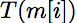But only the locations of the stores are keys in `t_hash`.

Now that we have `T`, the implementation of the rest of the algorithm is really simple:

``````def dept_store(m, p, k)
t_hash = {m => p}

(1..m.length-1).to_a.each do |j|
b = m[j] - k
t_hash[m[j]] = p[j] + T(b, m, t_hash)
end

t_hash.values.max
end``````

We first start out knowing thatand we add that information to `t_hash`. Then, we simply apply the relationrepeatedly. At the end, we simply return the maximum profit we can have!

Take home points:

• We broke down a complicated problem into simpler, smaller problems
• We used a function to keep track of state (in fact, we implicitly did the same for the earlier example; bonus points if you can define the function!)
• We came up with a relation that allowed us to “build up” the solution to our larger problem
• Our solution operates in linear time
• The same trick of dividing problem “based on where they end” was used quite effectively

## That’s All Folks!

Hopefully this article gave you some idea of the concepts behind dynamic programming
as well as some idea of how to implement them in Ruby.### Dhaivat Pandya

I'm a developer, math enthusiast and student.

#### New books out now!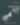Learn how Git works, and how to use it to streamline your workflow!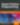Google, Netflix and ILM are Python users. Maybe you should too?